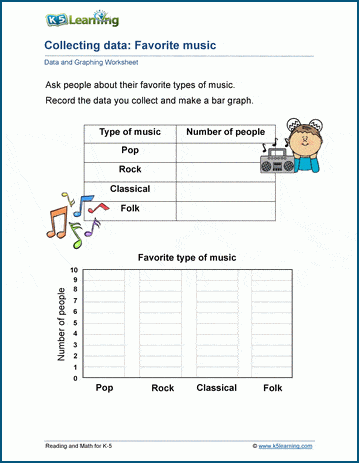# Data and Graphing Worksheets for Grade 2 Students

Our newly added data and graphing worksheets for grade 2 students focus on using graphs to represent data. With these worksheets they practice creating and reading pictographs, drawing line plots and bar graphs, depicting pie charts, line graphs and Venn diagrams.

## Sort and count

Students sort items into groups in these worksheets.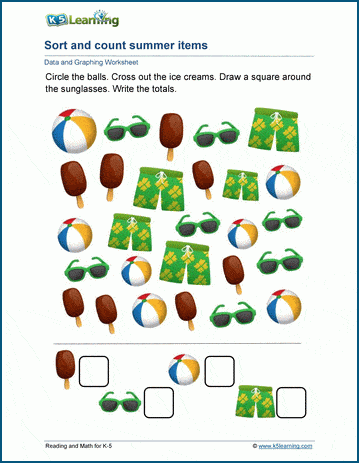## Tally sheets

Using tallies, students count and sort items.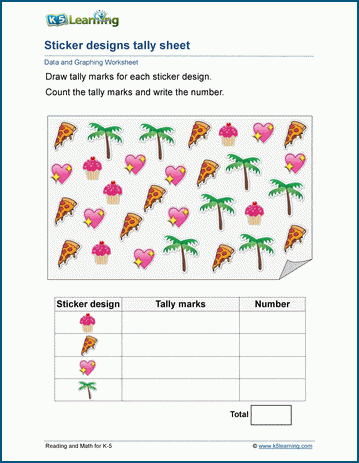## Pictographs

In these worksheets, students use pictographs and scaled pictographs to interpret data.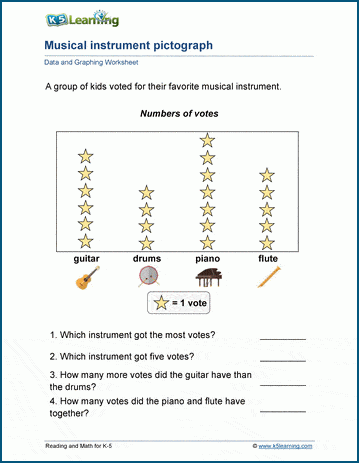## Line plots

Students practice creating line plots.## Bar graphs

Next, students work on bar charts using given data.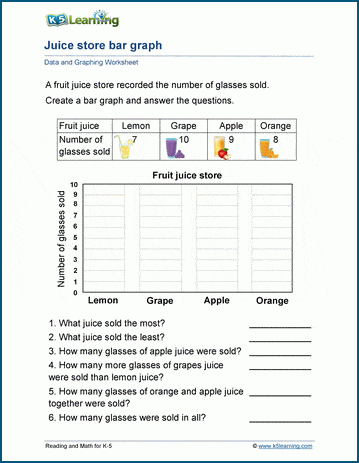## Analyzing bar graphs

Then, they turn to analyzing bar graphs.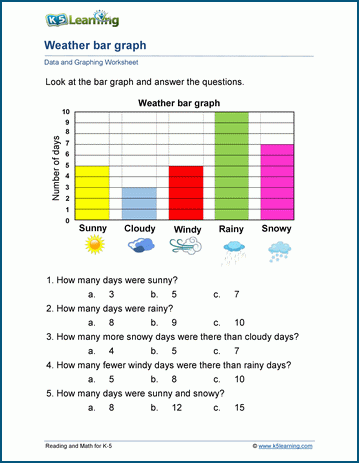## Creating pie charts

Pie charts display data in slices of a circle. Here students practice creating pie charts.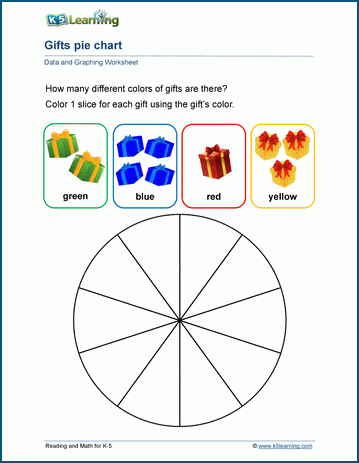## Reading pie charts

Once they’ve learned how to create pie charts, they practice analyzing data presented in pie charts.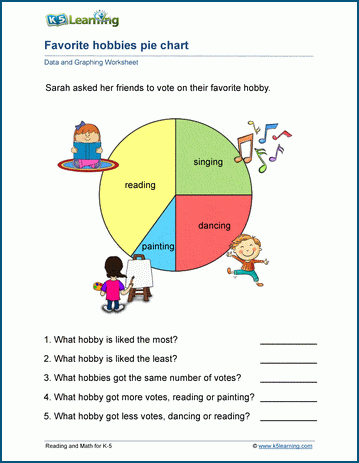## Venn diagrams

Venn diagrams are used to categorize items that have things in common and that are different. In these worksheets, students categorize items accordingly.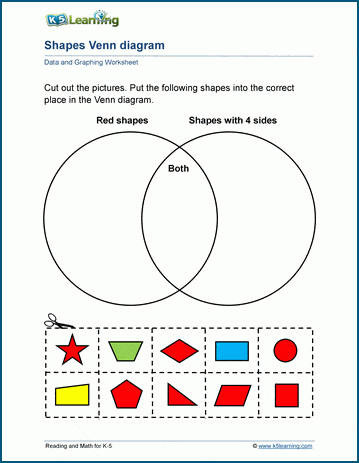## Line graphs

Students practice reading and drawing line graphs.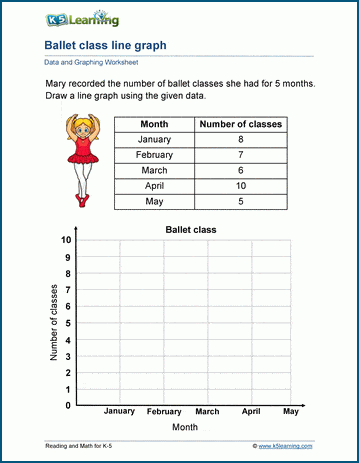## Data collection

Students collect real-world data, and write that data in bar charts and line graphs.